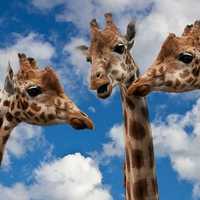# Pre-IB Weekly Quiz 1This page contains your first weekly quiz! Each week of July and August a new quiz will be published, so that you can make sure you are ready to start the Maths AI course in September with confidence.

Good luck and let us know how you got on!

1

If $$\frac { 5 }{ { a }^{ 4 } } \times { 3a }^{ 8 }=m{ a }^{ n }$$, what are the values of $$m$$ and $$n$$?

m =

n =

$$\frac { 5 }{ { a }^{ 4 } } \times { 3a }^{ 8 }=5{ a }^{ -4 }\times { 3a }^{ 8 }=15{ a }^{ -4+8 }=15{ a }^{ 4 }$$
2

Consider the linear model, f(x) = 3.56x -1.7, what is the value of x when f(x) = 69.5?

Substitute 69.5 into your equation and rearrange to solve, or input y = 3.56x - 1.7 in to your GDC and read from the table.

3

Which of the following is 6504905 correctly rounded to 3 significant figures

6500000 - the third non zero digit is 0, which stays the same because of the 4 that follows

4

What is the distance between coordinates A and B?

A: (3,5)

B:(5, 7)

Use Pythagoras to calculate this:

$$\sqrt{\left(\left(5-3\right)^{2}+\left(7-5\right)^{2}\right)}=2.828...$$

which rounds to 3sf to 2.83

5

Rearrange the equation $$y=3x+4$$ to make $$x$$ the subject

x=

6

A linear function has a y - intercept of 2.5 and a gradient of 1.5, which of the following is it?

7

a) What is the volume of the cuboid in the diagram below?

b) What is its surface area?Write a number only

a) Volume = cm3

b) Surface area = cm2

The volume of the base is 5 x 2, then multiply by the height, 6, giveives volume = 5 x 2 x 6

b) 2 faces are 5 x 2, 2 faces are 6 x 2 and 2 faces are 5 x 6, giving a total of 20 + 24 + 60 = 104cm2